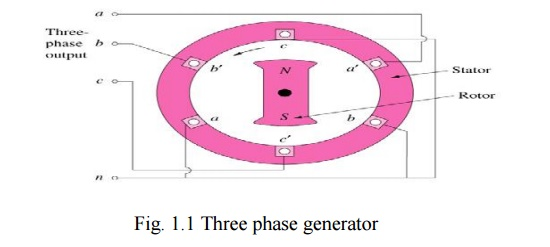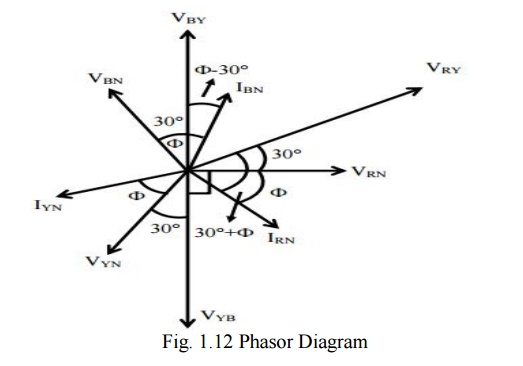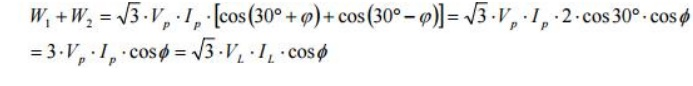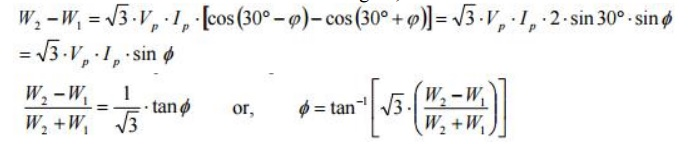Home | | Electrical Engineering and Instrumentation | Three Phase Circuits, A Review

# Three Phase Circuits, A Review

A generator consists of three coils placed 120° apart. The voltage generated are equal in magnitude but, out of phase by 120°. Three phase is the most economical polyphase system.

Three Phase Circuits, A Review

## Polyphase System

Circuit or system in which AC sources operate at the same frequency but different phases are known as polyphase.

## Two Phase System

A generator consists of two coils placed perpendicular to each other. The voltage generated by one lags the other by 90°.

## Three Phase System

A generator consists of three coils placed 120° apart. The voltage generated are equal in magnitude but, out of phase by 120°. Three phase is the most economical polyphase system.

### Importance of Three Phase System

Uniform power transmission and less vibration of three phase machines.

The instantaneous power in a 3f system can be constant (not pulsating).

High power motors prefer a steady torque especially one created by a rotating magnetic field.

Three phase system is more economical than the single phase. The amount of wire required for a three phase system is less than required for an equivalent single phase system.

## Three Phase Generation### Working

The generator consists of a rotating magnet (rotor) surrounded by a stationary  winding (stator).

Three separate windings or coils with terminals a-a’, b-b’, and c-c’ are physically placed 120° apart around the stator.

As the rotor rotates, its magnetic field cuts the flux from the three coils and induces voltages in the coils.

The induced voltage have equal magnitude but out of phase by 120°.

### Three-Phase Waveform### Three Phase Quantities

#### Balanced 3f Voltages

Balanced three phase voltages:

Same magnitude (VM )

120° phase shift#### Balanced 3f Currents

Balanced three phase currents:

Same magnitude (IM )

120° phase shift### Phase Sequence

#### Positive Sequence#### Negative Sequence## POWER

The instantaneous power is constant.

p(t)= pa(t)+pb(t)+ pc(t)

= 3 [VMIM /2] cos(q)

= 3VrmsIrmscos(q )

## Three Phase Connection### WYE Connection### Balanced Y-Y Connection## Phase Currents and Line Currents

In Y-Y system, line current is equal to phase current, IL = IP

### Delta Connected Sources### Balanced D- D Connection## PHASE VOLTAGE AND LINE VOLTAGE

In D-D system, line voltages are equal to phase voltages: VL = VP

Power Measurement in a Three Phase Circuit Using Two-Wattmeter Method## Phasor DiagramThe reading of the first wattmeter is,The reading of the second wattmeter is,The sum of the two wattmeter readings is,The difference of the two wattmeter readings is,Power factor (cosф) can be determined using the above expression.

Study Material, Lecturing Notes, Assignment, Reference, Wiki description explanation, brief detail
Electrical Engineering and Instrumentation : DC Machines : Three Phase Circuits, A Review |